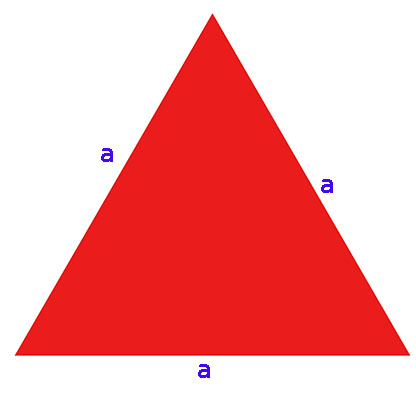# O = a + b +c

Legend:

## c – the length of the side of the triangle

Calculate the perimeter of the triangle with the base a = 10 cm, side b = 9 cm and side c = 8 cm

#### O = 27 cm

The perimeter of a triangle with a base a = 10 cm, side b = 9 cm and side c = 8 cm, is 27cm.

# The formula for the perimeter of an equilateral triangle# O = a + b +c

calculating the perimeter of an equilateral triangle, which has all sides of equal length. If we know the side length of an equilateral triangle, we can write it down, that his circuit is equal: